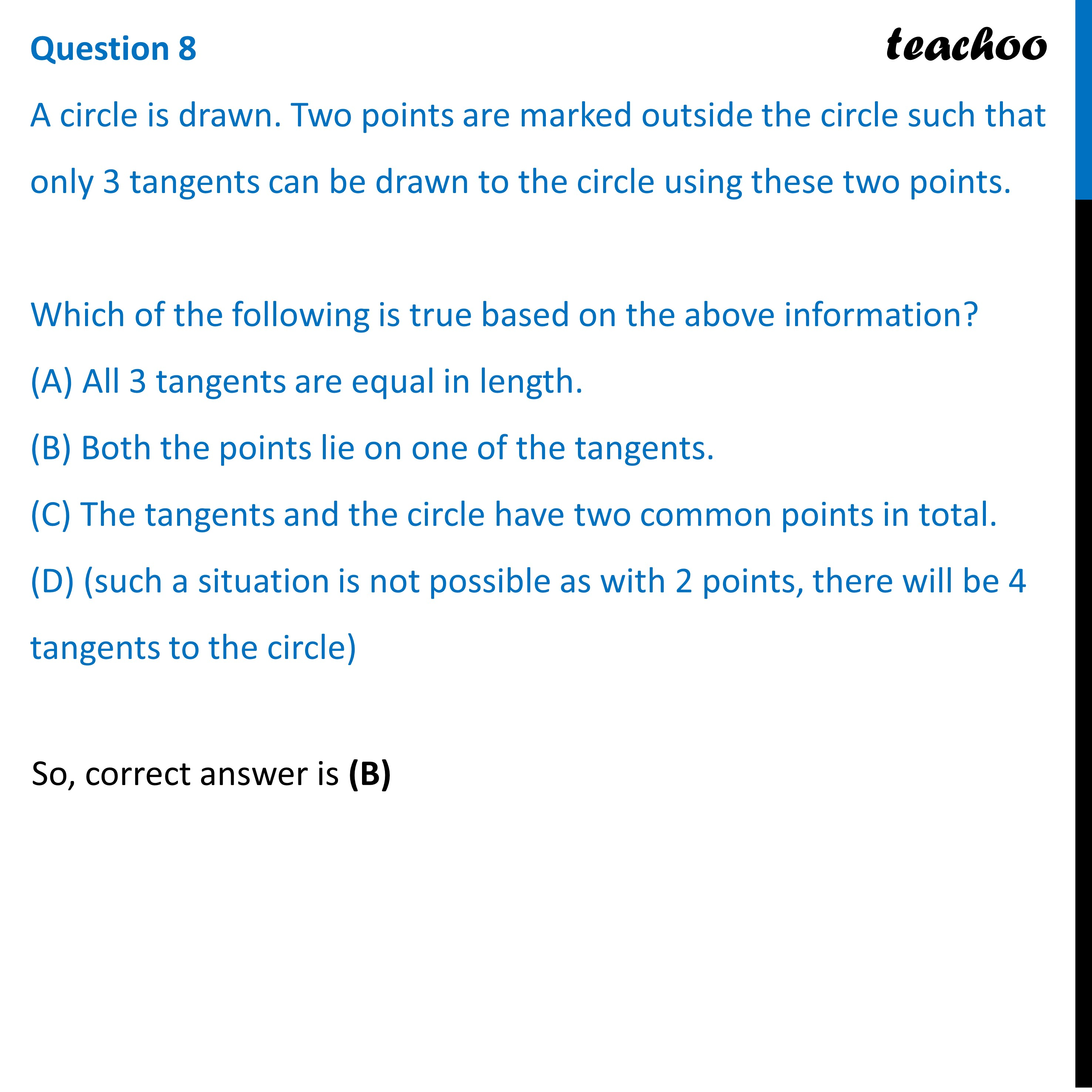Practice Questions CBSE - Maths Class 10 (2023 Boards)

Class 10
Solutions of Sample Papers for Class 10 Boards

## (D) (such a situation is not possible as with 2 points, there will be 4 tangents to the circle)Learn in your speed, with individual attention - Teachoo Maths 1-on-1 Class

### Transcript

A circle is drawn. Two points are marked outside the circle such that only 3 tangents can be drawn to the circle using these two points. Which of the following is true based on the above information? (A) All 3 tangents are equal in length. (B) Both the points lie on one of the tangents. (C) The tangents and the circle have two common points in total. (D) (such a situation is not possible as with 2 points, there will be 4 tangents to the circle)